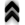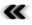### 3.2 Mathematics and Algorithms

While the machines constructed to perform computational tasks undoubtedly are capable of addressing mathematical problems, it requires special adaptations in order to carry mathematical procedures from the purely theoretical settings to the machine world. The declarative parts of mathematical formalisms pose a problem for the implementation of algorithms. This is true even for very simple mathematical statements such as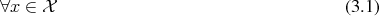which may be encountered for example in preconditions. In the setting of mathematics the statement in itself is suﬃcient, however, for an implementation some kind of enforcement is required, if it is not to be just silently assumed. A practical diﬃculty arises from the fact that the mathematical expression is timeless and does not consume any resources at all beside itself. On the digital computer, however, memory consumption and computing requirements are always a limiting factor.

The limited nature of the computing world also becomes apparent, when comparing the limited numerical data types available to the machine, which may require special attention from the programmer, to the plethora of sets a mathematician has at his command.

A very simple example concerns integers which are commonly denoted as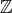in mathematics. While programming languages oﬀer a data type of exactly the same name, it falls far short from the mathematical entity, with which it shares its name. The most apparent fact is that the data type encompasses only a ﬁnite amount of distinct elements, which directly corresponds to the amount of memory it requires, while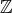is inﬁnite and has no resource consumption associated with it. Furthermore, while both cases are groups, the topology of integer data types resembles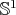, as shown in the left part of Figure 3.5 where the minimum and maximum values are next to each other and wrap around, which substantially deviates from the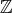topology.

In the case of ﬂoating point data types, such as deﬁned by IEEE 754 , which are used to emulate the mathematical concepts of the rational numbersand the real numbers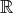, are at best capable of exactly representing a subset of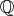, but fail to capture irrational numbers at all. While the topology of this data type is linear for the numbers it represents, there is also an isolated element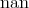, as is depicted in the right half of Figure 3.5. Furthermore, while being a super set of the integer data type, it fails to be a group.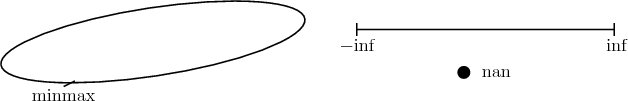Figure 3.5: Diﬀerent topologies inherent to data types. The data type on the left wraps around from its maximum to minimum values, corresponding to an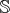type topology. The data type on the right even includes an isolated point (nan).

While being far from comprehensive, the given examples should indicate that care has to be taken, when considering implementing algorithms on a digital computer. An awareness of a machine’s limitations, which may vary considerably due to architecture and platform, is essential for realizing reliable scientiﬁc computing applications.